StatLect

# Logistic classification model - Maximum likelihood estimation

This lecture deals with maximum likelihood estimation of the logistic classification model (also called logit model or logistic regression).

Before reading this lecture, you might want to revise the lectures on maximum likelihood estimation and on the logit model.## Model and notation

Remember that in the logit model the output variableis a Bernoulli random variable (it can take only two values, either 1 or 0) andwhereis the logistic function,is avector of inputs andis avector of coefficients.

Furthermore,The vector of coefficientsis the parameter to be estimated by maximum likelihood.

We assume that the estimation is carried out with an IID sample comprisingdata points## The likelihood

The likelihood of an observationcan be written asIf you are wondering about the exponentsandor, more in general, about this formula for the likelihood, you are advised to revise the lecture on Classification models and their maximum likelihood estimation.

Denote thevector of all outputs byand thematrix of all inputs by. Since the observations are IID, then the likelihood of the entire sample is equal to the product of the likelihoods of the single observations:## The log-likelihood

The log-likelihood of the logistic model isProof

It is computed as follows: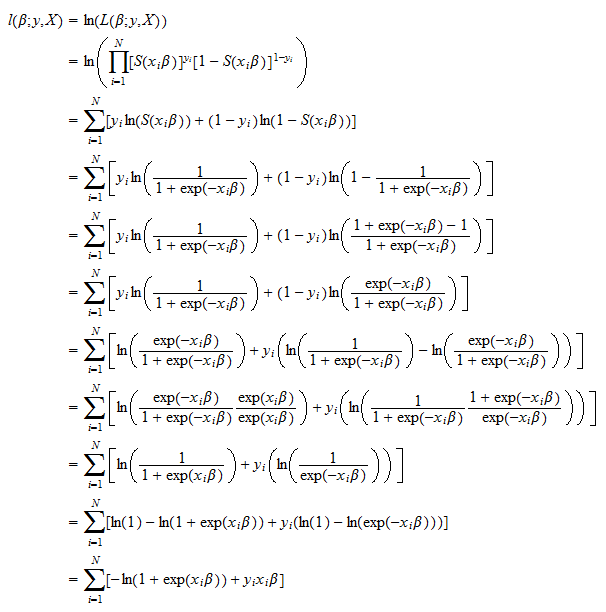## The score

The score vector, that is the vector of first derivatives of the log-likelihood with respect to the parameter, isProof

This is obtained as follows: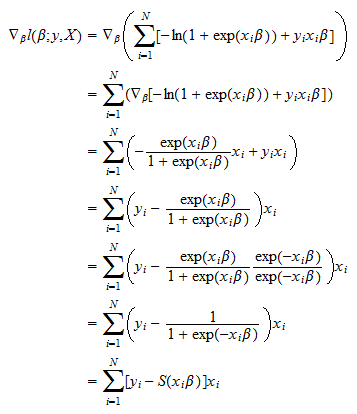## The Hessian

The Hessian, that is the matrix of second derivatives, isProof

It can be proved as follows: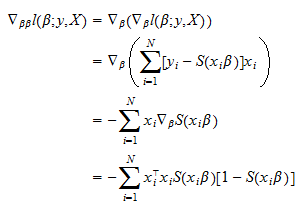where we have used the fact that the derivative of the logistic functionis## The first-order condition

The maximum likelihood estimatorof the parametersolvesIn general, there is no analytical solution of this maximization problem and a solution must be found numerically (see the lecture entitled Maximum likelihood algorithm for an introduction to the numerical maximization of the likelihood).

Moreover, this maximization problem is not guaranteed to have a solution because some pathological situations can arise in which the log-likelihood is an unbounded function of the parameters. In these situations the log-likelihood can be made as large as desired by appropriately choosing. This happens when the residuals can be made as small as desired (so-called perfect separation of classes). It is not a common situation. It means that the model can perfectly fit the observed classes. In all other situations, the maximization problem has a solution, and at the maximum the score vector satisfies the first order conditionthat is,Note thatis the error committed by usingas a predictor of. It is similar to a regression residual (see Linear regression). Furthermore the first order condition above is similar to the first order condition that is found when estimating a linear regression model by ordinary least squares: it says that the residuals need to be orthogonal to the predictors.

## Newton-Raphson method

The first order condition above has no explicit solution. In most statistical software packages it is solved by using the Newton-Raphson method. The method is pretty simple: we start from a guess of the solution(e.g.,), and then we recursively update the guess with the equation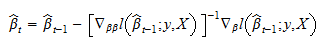until numerical convergence (ofto the solution).

Denote bythevector of conditional probabilities of the outputs computed by usingas parameter:Denote bythediagonal matrix (i.e., having all off-diagonal elements equal to) such that the elements on its diagonal are, ...,: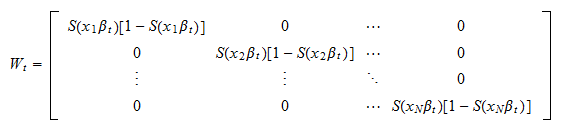Thematrix of inputswhich is called design matrix (as in linear regression), is assumed to be a full-rank matrix.

By using this notation, the score in Newton-Raphson recursive formula can be written asand the Hessian asTherefore, the Newton-Raphson formula becomeswhere the existence of the inverseis guaranteed by the assumption thathas full-rank (the assumption also guarantees that the log-likelihood is concave and the maximum likelihood problem has a unique solution).

## Iteratively reweighted least squares

If you deal with logit models, you will often read that they can be estimated by Iteratively Reweighted Least Squares (IRLS). The Newton-Raphson formula above is equivalent to the IRLS formula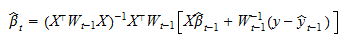that is obtained by performing a Weighted Least Squares (WLS) estimation with weightsof a linear regression of the dependent variableson the regressors.

Proof

WriteasThen, we can re-write the Newton Raphson formula as follows: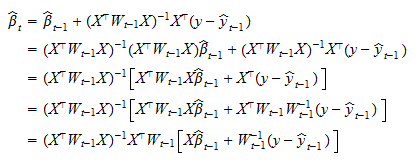The IRLS formula can alternatively be written as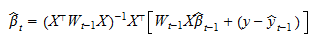## Covariance matrix of the estimator

The asymptotic covariance matrix of the maximum likelihood estimatoris usually estimated with the Hessian (see the lecture on the covariance matrix of MLE estimators), as follows: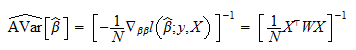whereand(is the last step of the iterative procedure used to maximize the likelihood). As a consequence, the distribution ofcan be approximated by a normal distribution with mean equal to the true parameter value and variance equal toThe book

Most of the learning materials found on this website are now available in a traditional textbook format.

Glossary entries
Share